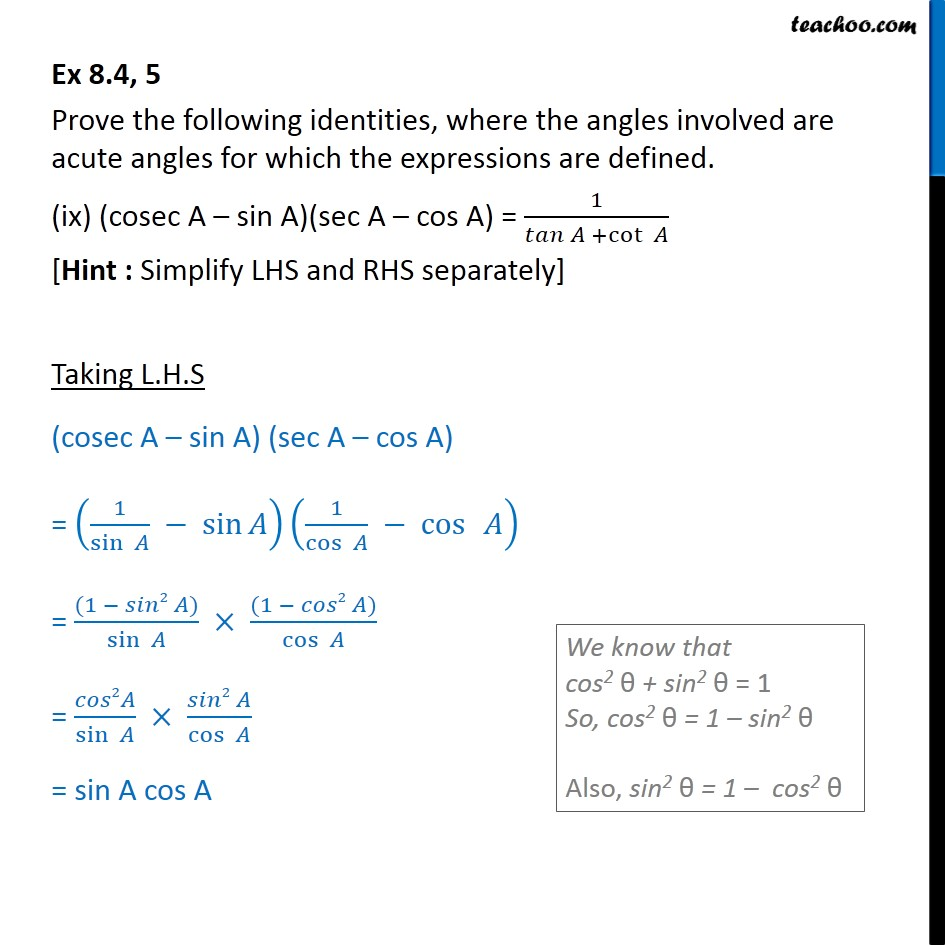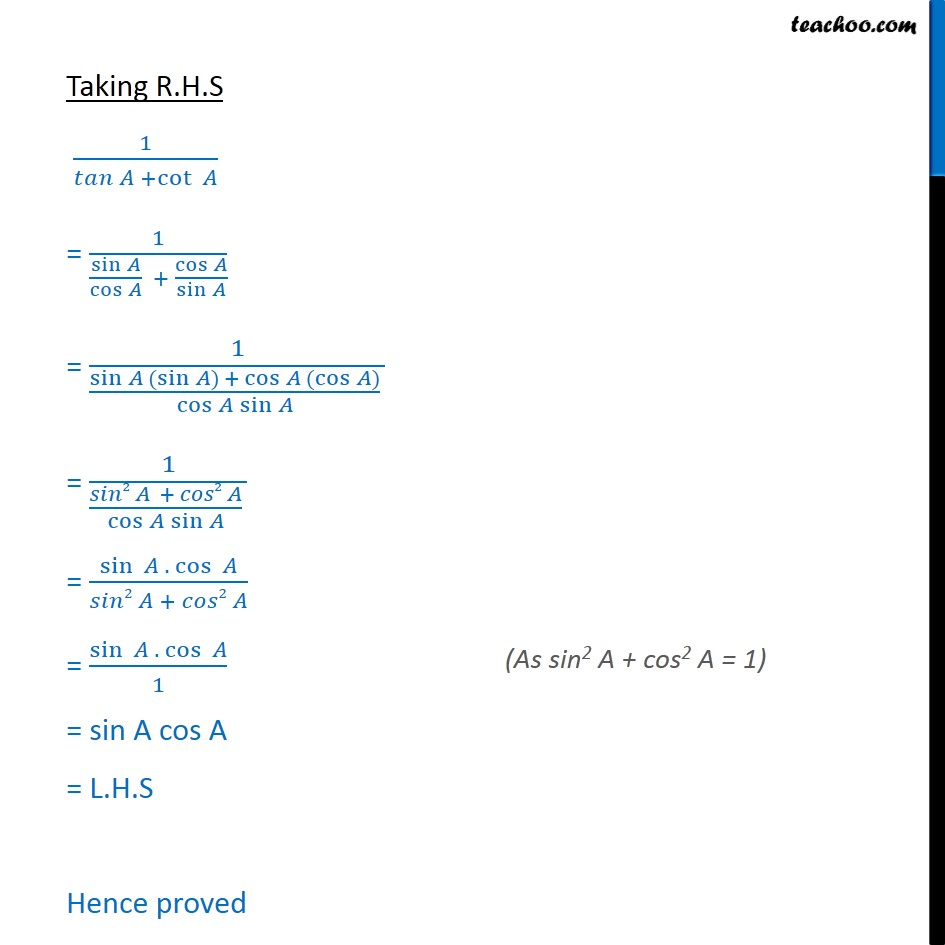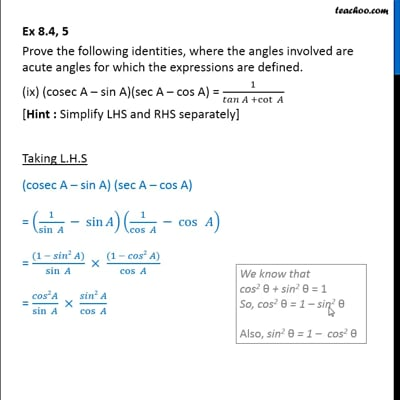Ex 8.4

Chapter 8 Class 10 Introduction to Trignometry
Serial order wiseThis video is only available for Teachoo black users

Get live Maths 1-on-1 Classs - Class 6 to 12

### Transcript

Ex 8.4, 5 Prove the following identities, where the angles involved are acute angles for which the expressions are defined. (ix) (cosec A sin A)(sec A cos A) = 1/( +cot ) [Hint : Simplify LHS and RHS separately] Taking L.H.S (cosec A sin A) (sec A cos A) = (1/sin sin )(1/cos cos ) = ((1 2 ))/sin ((1 2 ))/cos = 2 /sin ( 2 )/cos = sin A cos A Taking R.H.S 1/( +cot ) = 1/(sin /cos + cos /sin ) = 1/(sin (sin ) + cos (cos ) /cos sin ) = 1/(( 2 + 2 )/cos sin ) = sin . cos /( 2 + 2 ) = sin . cos /1 = sin A cos A = L.H.S Hence proved